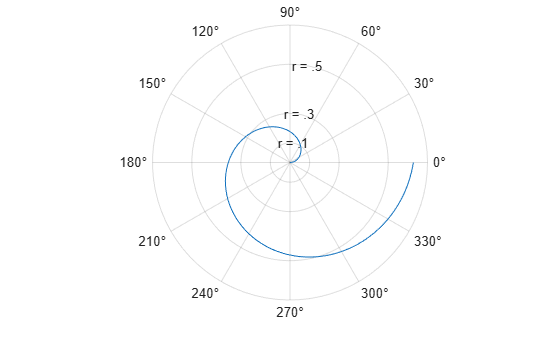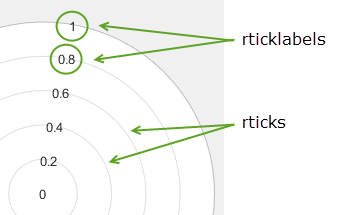# rticklabels

## 语法

rticklabels(labels)
rl = rticklabels
rticklabels('auto')
rticklabels('manual')
m = rticklabels('mode')
___ = rticklabels(pax,___)

## 说明

rticklabels(labels) 设置当前坐标区的 r 轴刻度标签。可将 labels 指定为字符串数组或字符向量元胞数组，例如 {'January','February','March'}。如果您指定标签，则 r 轴刻度值和刻度标签不会再根据对坐标区所做的更改而自动更新。
rl = rticklabels 返回当前坐标区的 r 轴刻度标签。

rticklabels('auto') 设置自动模式，从而允许坐标区确定 r 轴刻度标签。如果您设置了标签，然后又想将它们设置回默认值，则可以使用此选项。
rticklabels('manual') 设置手动模式，从而将 r 轴刻度标签冻结在当前值。
m = rticklabels('mode') 返回 r 轴刻度标签模式的当前值，即 'auto' 或 'manual'。默认情况下，该模式为自动，除非您指定刻度标签或将模式设置为手动。

___ = rticklabels(pax,___) 使用 pax 指定的坐标区，而不是使用当前坐标区。指定 ax 作为上述任何语法的第一个输入参数。

## 示例

theta = linspace(0,2*pi); rho = theta/10; polarplot(theta,rho) rticks([0.1 0.25 0.5]) rticklabels({'r = .1','r = .3','r = .5'})theta = 0:0.01:2*pi; rho = 50*sin(2*theta); polarplot(theta,rho) pax = gca; rticks(pax,[10 25 50]) rticklabels(pax,{'r = 10','r = 25','r = 50'})theta = linspace(0,2*pi); rho = theta/10; polarplot(theta,rho) rticks([0.1 0.25 0.5]) rticklabels({'r = .1','r = .3','r = .5'})rticks('auto') rticklabels('auto')theta = 0:0.01:2*pi; rho = 50*sin(2*theta); polarplot(theta,rho) rticklabels({})## 输入参数

• 要指定刻度值，请使用 rticks 函数。

• 如果没有为所有刻度值指定足够的标签，则 MATLAB® 会为其余的刻度使用空字符向量标签。

• 如果将刻度标签指定为分类数组，MATLAB 将使用数组中的值，而不是类别。

## 输出参数

• 'auto' - 坐标区自动确定 r 轴刻度标签。

• 'manual' - 坐标区使用手动指定的 r 轴刻度标签。

## 详细信息

### 刻度标签## 算法

rticklabels 函数可设置和查询与 r 轴刻度标签相关的多个极坐标区属性。

• RTickLabel - 存储 r 轴刻度标签文本的属性。

• RTickLabelMode - 存储 r 轴刻度标签模式的属性。当您使用 rticklabels 设置 r 轴刻度标签时，此属性将更改为 'manual'

• RTickMode - 存储 r 轴刻度值模式的属性。当您使用 rticklabels 设置 r 轴刻度标签时，此属性将更改为 'manual'